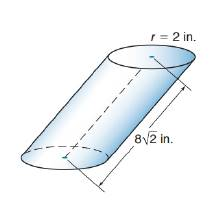Chapter 9.3, Problem 13E### Elementary Geometry for College St...

6th Edition
Daniel C. Alexander + 1 other
ISBN: 9781285195698

#### Solutions

Chapter
Section### Elementary Geometry for College St...

6th Edition
Daniel C. Alexander + 1 other
ISBN: 9781285195698
Textbook Problem
4 views

# Find the volume of the oblique circular cylinder. The axis meets the plane of the base to form a 45 ∘ angle.To determine

To calculate:

The volume of the oblique circular cylinder.

Explanation

Given:

The oblique circular cylinder has the indicated dimensions. The axis meets the plane of the base to form a 45 angle.

Formula used:

The volume of the right circular cylinder is V=πr2h.

Calculation:

Since the axis meets the plane of the base to form an 45 angle, the axis and the altitude form a right triangle.

From the figure,

sin45=h8212=h82

### Still sussing out bartleby?

Check out a sample textbook solution.

See a sample solution

#### The Solution to Your Study Problems

Bartleby provides explanations to thousands of textbook problems written by our experts, many with advanced degrees!

Get Started

#### Simplify the expressions in Exercises 97106. (xy)1/3(yx)1/3

Finite Mathematics and Applied Calculus (MindTap Course List)

#### Find each limit. a. limx2| x2 |x2 b. limx2+| x2 |x2

Calculus: An Applied Approach (MindTap Course List)

#### Evaluate the limit. limx0e2xe2xln(x+1)

Single Variable Calculus: Early Transcendentals, Volume I

#### Graph: f(x)=x39x.

College Algebra (MindTap Course List)